Courses

Test: Median

10 Questions MCQ Test Quantitative Aptitude for GMAT | Test: Median

Description
Attempt Test: Median | 10 questions in 20 minutes | Mock test for GMAT preparation | Free important questions MCQ to study Quantitative Aptitude for GMAT for GMAT Exam | Download free PDF with solutions
QUESTION: 1

Solution:
QUESTION: 2

Solution:
QUESTION: 3

T is a set of y integers, where 0 < y < 7. If the average of Set T is the positive integer x, which of thefollowing could NOT be the median of Set T?

Solution:

The possible values of (2/7)y are as follows: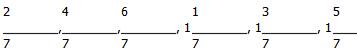However, the median of a set of integers must always be either an integer or a fraction with a denominator of 2 (e.g. 2.5, or 5/2). So (2/7)y cannot be the median of Set T.  The correct answer is E.

QUESTION: 4

a, b, and c are integers and a < b < c. S is the set of all integers from a to b, inclusive. Q is the setof all integers from b to c, inclusive. The median of set S is (3/4) b. The median of set Q is (7/8) c.If R is the set of all integers from a to c, inclusive, what fraction of c is the median of set R?

Solution:

Since S contains only consecutive integers, its median is the average of the extreme values a and b.  We also know that the median of S is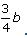We can set up and simplify the following equation: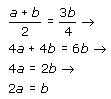Since set Q contains only consecutive integers, its median is also the average of the extreme values, in this case b and c.  We also know that the median of Q is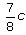. We can set up and simplify the following equation: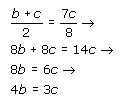We can find the ratio of a to c as follows: Taking the first equation, 2a = b → 8a= 4b and the second equation, 4b=3c and setting them equal to each other, yields the following: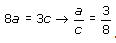Since set R contains only consecutive integers, its median is the average of the extreme values a and c: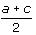. We can use the ratio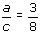to substitute 3c/8 for a: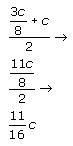Thus the median of set R is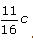The correct answer is C

QUESTION: 5

Jim Broke’s only source of income comes from his job as a question writer. In this capacity, Jim earnsa flat salary of \$200 per week plus a fee of \$9 for every question that he writes. Every year, Jim takesexactly two weeks of unpaid vacation to visit his uncle, a monk in Tibet, and get inspired for the nextyear. If a regular year consists of 52 weeks and the number of questions that Jim wrote in each ofthe past 5 years was an odd number greater than 20, which of the following could be Jim’s medianannual income over the past 5 years?

Solution:

Since a regular year consists of 52 weeks and Jim takes exactly two weeks of unpaid vacation, he works for a total of 50 weeks per year. His flat salary for a 50-week period equals 50 × \$200 = \$10,000 per year. Because the number of years in a 5-year period is odd, Jim’s median income will coincide with his annual income in one of the 5 years. Since in each of the past 5 years the number of questions Jim wrote was an odd number greater than 20, his commission compensation above the flat salary must be an odd multiple of 9. Subtracting the \$10,000 flat salary from each of the answer choices, will result in the amount of commission. The only odd values are \$15,673, \$18,423 and \$21,227 for answer choices B, D, and E, respectively. Since the total amount of commission must be divisible by 9, we can analyze each of these commission amounts for divisibility by 9. One easy way to determine whether a number is divisible by 9 is to sum the digits of the number and see if this sum is divisible by 9. This analysis yields that only \$18,423 (sum of the digits = 18) is divisible by 9 and can be Jim’s commission.  Hence, \$28,423 could be Jim’s median annual income. The correct answer is choice D.

QUESTION: 6

During a behavioral experiment in a psychology class, each student is asked to compute his or herlucky number by raising 7 to the power of the student's favorite day of the week (numbered 1through 7 for Monday through Sunday respectively), multiplying the result by 3, and adding this tothe doubled age of the student in years, rounded to the nearest year. If a class consists of 28students, what is the probability that the median lucky number in the class will be a non-integer?

Solution:

Since any power of 7 is odd, the product of this power and 3 will always be odd.  Adding this odd number to the doubled age of the student (an even number, since it is the product of 2 and some integer) will always yield an odd integer. Therefore, all lucky numbers in the class will be odd.

The results of the experiment will yield a set of 28 odd integers, whose median will be the average of the 14th and 15th greatest integers in the set. Since both of these integers will be odd, their sum will always be even and their average will always be an integer. Therefore, the probability that the median lucky number will be a non-integer is 0%.

QUESTION: 7

For the set of terms [x, y, x + y, x – 4y, xy, 2y], if y > 6 and the mean of the set equals y + 3, then
the median must be

Solution:

The mean of a set is equal to the sum of terms divided by the number of terms in the set. Therefore,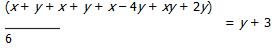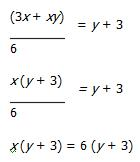x = 6. Given that y > 6 and substituting x = 6, the terms of the set can now be ordered from least to greatest:
6 – 4y, 6, y, y + 6, 2y, 6y. The median of a set of six terms is the mean of the third and fourth terms (the two middle terms). The mean of the terms y and y + 6 is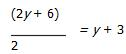QUESTION: 8

Set A: 3, x, 8, 10 Set B: 4, y, 9, 11. The terms of each set above are given in ascending order. If the median of Set A is equal to the median of Set B, what is the value of y – x?

Solution:

Since each set has an even number of terms, the median of each set will be equal to the average of the two middle terms of that set. So, the median of Set A will be equal to the average of x and 8. The median of Set B will be equal to the average of y and 9. The question tells us that the median of Set A is equal to the median of Set B. We can express this algebraically as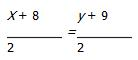We can multiply both sides by 2:
x + 8 = y + 9

We can subtract x from both sides (remember, we are looking for y – x):
We can multiply both sides by 2:
x + 8 = y + 9

We can subtract x from both sides (remember, we are looking for yx):

8 = y – x + 9

We can subtract 9 from both sides to isolate y – x:
y – x = 8 – 9 = -1

QUESTION: 9

Set S includes elements {8, 2, 11, x, 3, y} and has a mean of 7 and a median of 5.5. If x < y, then
which of the following is the maximum possible value of x?

Solution:

To find the maximum possible value of x, we'll first consider that the set's mean is 7, and then that its median is 5.5.

For any set, the sum of the elements equals the mean times the number of elements. In this case, the mean is 7 and the number of elements is 6, so the sum of the elements equals 42.

42 = 8 + 2 + 11 + x + 3 + y
42 = 24 + x + y
18 = x + y

Now consider that the median is 5.5. Letting x = 1 and y = 17 such that they sum to 18, we can arrange the values in increasing order as follows:

x, 2, 3, 8, 11, y

Since 3 and 8 are the middle values, the median equals 5.5 as required. The question asks for the maximal value of x, so let's increase x as far as possible without changing the median. As x increases to 3 (and y decreases to 15), the middle values of 3 and 8 don't change, so the median remains at 5.5. However, as x increases beyond 3, the median also increases, so the maximal value of x that leaves the median at 5.5 is 3.

QUESTION: 10

The temperatures in Celsius recorder at 6 in the morning in various parts of a certain country were
10,5,-2,-1,-5 and 15. What is the median of these temperatures?

Solution:

First, we arrange the 10,5,-2,-1,-5 and 15 at sequence: -5, -2, -1, 5, 10, 15. So, the median is (-1+5)/2=2Use Code STAYHOME200 and get INR 200 additional OFF Use Coupon Code

Track your progress, build streaks, highlight & save important lessons and more!

Similar ContentRelated tests